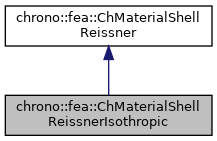chrono::fea::ChMaterialShellReissnerIsothropic Class Reference

## Description

For backward compatibility only! New approach: create a ChElasticityReissnerOrthotropic and create a ChMaterialShellReissner by passing the elasticity as a parameter.

#include <ChMaterialShellReissner.h>

Inheritance diagram for chrono::fea::ChMaterialShellReissnerIsothropic:[legend]
Collaboration diagram for chrono::fea::ChMaterialShellReissnerIsothropic:[legend]

## Public Member Functions

ChMaterialShellReissnerIsothropic (double mdensity, double E, double nu, double alpha=1.0, double beta=0.1)
Construct an isotropic material. More...Public Member Functions inherited from chrono::fea::ChMaterialShellReissner
ChMaterialShellReissner (std::shared_ptr< ChElasticityReissner > melasticity)

ChMaterialShellReissner (std::shared_ptr< ChElasticityReissner > melasticity, std::shared_ptr< ChPlasticityReissner > mplasticity)

ChMaterialShellReissner (std::shared_ptr< ChElasticityReissner > melasticity, std::shared_ptr< ChPlasticityReissner > mplasticity, std::shared_ptr< ChDampingReissner > mdamping)

virtual void ComputeStress (ChVector<> &n_u, ChVector<> &n_v, ChVector<> &m_u, ChVector<> &m_v, const ChVector<> &eps_u, const ChVector<> &eps_v, const ChVector<> &kur_u, const ChVector<> &kur_v, const double z_inf, const double z_sup, const double angle, ChShellReissnerInternalData *mdata_new=nullptr, const ChShellReissnerInternalData *mdata=nullptr)
Compute the generalized cut force and cut torque, given the actual generalized section strain expressed as deformation vector e and curvature k, that is: {n_u,n_v,m_u,m_v}=f({e_u,e_v,k_u,k_v}), and given the actual material state required for plasticity if any (but if mdata=nullptr, computes only the elastic force). More...

virtual void ComputeStiffnessMatrix (ChMatrixRef K, const ChVector<> &eps_u, const ChVector<> &eps_v, const ChVector<> &kur_u, const ChVector<> &kur_v, const double z_inf, const double z_sup, const double angle, const ChShellReissnerInternalData *mdata=nullptr)
Compute the 6x6 tangent material stiffness matrix [Km] = dσ/dε at a given strain state, and at given internal data state (if mdata=nullptr, computes only the elastic tangent stiffenss, regardless of plasticity). More...

void SetElasticity (std::shared_ptr< ChElasticityReissner > melasticity)
Set the elasticity model for this section. More...

std::shared_ptr< ChElasticityReissnerGetElasticity ()
Get the elasticity model for this section. More...

void SetPlasticity (std::shared_ptr< ChPlasticityReissner > mplasticity)
Set the plasticity model for this section. More...

std::shared_ptr< ChPlasticityReissnerGetPlasticity ()
Get the elasticity model for this section, if any. More...

void SetDamping (std::shared_ptr< ChDampingReissner > mdamping)
Set the damping model for this section. More...

std::shared_ptr< ChDampingReissnerGetDamping ()
Get the damping model for this section. More...

void SetDensity (double md)
Set the density of the shell (kg/m^3)

double GetDensity () const

double Get_rho () const
for backward compatibility - use GetDensity instead

## ◆ ChMaterialShellReissnerIsothropic()

 chrono::fea::ChMaterialShellReissnerIsothropic::ChMaterialShellReissnerIsothropic ( double mdensity, double E, double nu, double alpha = 1.0, double beta = 0.1 )
inline

Construct an isotropic material.

Parameters
 mdensity material density E Young's modulus nu Poisson ratio alpha shear factor beta torque factor

The documentation for this class was generated from the following file:
• /builds/uwsbel/chrono/src/chrono/fea/ChMaterialShellReissner.h